# 5. Getting Spatial with sftrack

library("sftrack")
# Make tracks from raw data
data('raccoon', package = 'sftrack')
raccoon$month <- as.POSIXlt(raccoon$timestamp)$mon+1 raccoon$time <- as.POSIXct(raccoon$timestamp, tz='EST') coords = c('longitude','latitude') group = list(id = raccoon$animal_id, month = as.POSIXlt(raccoon$timestamp)$mon+1)
time = 'time'
error = 'fix'
crs = '+proj=longlat +ellps=WGS84 +datum=WGS84 +no_defs'
my_sftrack <- as_sftrack(data = raccoon, coords = coords, group = group, time = time, error = error, crs = crs)
my_sftraj <- as_sftraj(data = raccoon, coords = coords, group = group, time = time, error = error, crs = crs)

## Geometry column

As stated earlier, the geometry column is built using sf, so the column functions exactly as it would in sf. You can modify it and redefine it using the sf tools. More specifically the geometry column of an sf_track object is an sfc column. The main difference between a standard sf object created using st_as_sf is that we automatically allow empty geometries, where as this option is turned off by default in st_as_sf().

my_sftrack$geometry ## Geometry set for 445 features (with 168 geometries empty) ## Geometry type: POINT ## Dimension: XY ## Bounding box: xmin: -80.28149 ymin: 26.06761 xmax: -80.27046 ymax: 26.07706 ## CRS: +proj=longlat +ellps=WGS84 +datum=WGS84 +no_defs ## First 5 geometries: ## POINT EMPTY ## POINT (-80.27906 26.06945) ## POINT EMPTY ## POINT EMPTY ## POINT (-80.27431 26.06769) An sftrack object is simply an sfc of sfc_POINTS, this contrasts with an sftraj object which is a mixture of a POINT and LINESTRING. This is because a trajectory can have a start point and an NA end point, a line segment, or an NA and an end point. This allows no-loss conversion back and forth between sftrack and an sftraj, and because linestrings can not have a NULL point in them. my_sftraj$geometry
## Geometry set for 445 features  (with 168 geometries empty)
## Geometry type: GEOMETRY
## Dimension:     XY
## Bounding box:  xmin: -80.28149 ymin: 26.06761 xmax: -80.27046 ymax: 26.07706
## CRS:           +proj=longlat +ellps=WGS84 +datum=WGS84 +no_defs
## First 5 geometries:
## POINT EMPTY
## POINT (-80.27906 26.06945)
## POINT EMPTY
## POINT EMPTY
## LINESTRING (-80.27431 26.06769, -80.2793 26.06867)

This does mean that not all sf functions will handle an sftraj object like it would an sftrack if there are NAs in the data set. In these cases st_is_empty() can help to subset the points that contain geometry data.

## Working with sf

As an sftrack object is an sf object essentially all of the sf functions will work on it.

library("sf")
st_length(my_sftraj)[1:10]
## Units: [m]
##     0.000000   0.000000   0.000000   0.000000 510.096380 107.395332
##     5.625847   0.000000   0.000000  15.748173
df1 <- data.frame(
id = c(1,1,1,1),
time = as.POSIXct('2020-01-01 12:00:00', tz = 'UTC') + 60*60*(1:4),
x = c(1,3,3,2),
y = c(1,1,3,4)
)

c(1,2),
c(5,2),
c(5,0)
)
)

animal1 <- as_sftraj(df1)
plot(animal1)

plot(road,  col = 'red', add = T)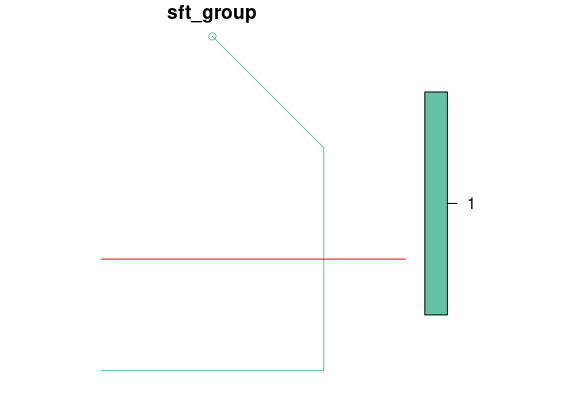# Does the animal cross the road?

any(st_intersects(animal1, road, sparse = F))
##  TRUE
# When?
active_group(my_sftraj$sft_group) ##  "id" plot(my_sftraj)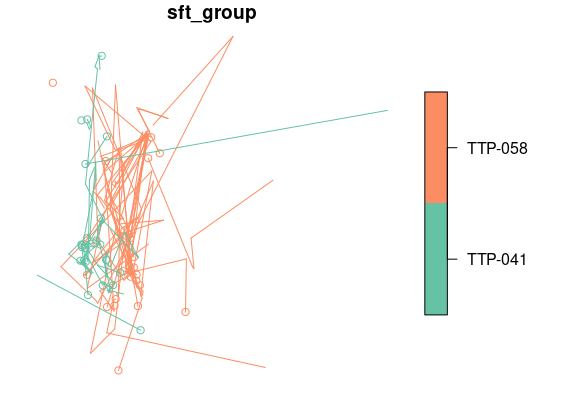Most arguments for plot.sf are available to use as additional arguments to plot. plot(my_sftrack, axes = TRUE, cex=5)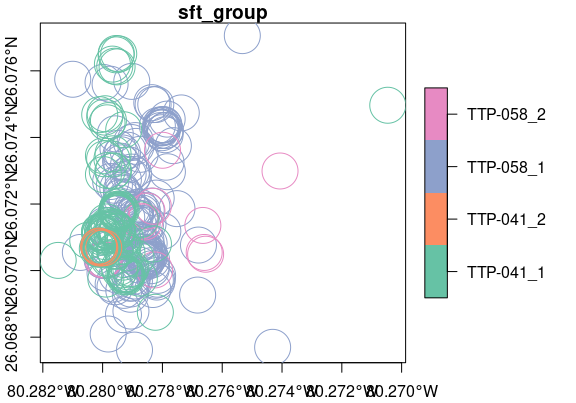### ggplot2 This is a work in progress, but theres a geom_sftrack function that feeds geom_sf with the correct plotting information. Like geom_sf you input data into the geom_sftrack function and not into ggplot(). Again ggplot assumes active_group is the grouping variable. Plots vary slightly based on if they’re sftrack of sftraj class. Geom_sftrack is essentially geom_sf(data = data, aes(color = group_labels(data))) with NULL points subetted out. This may help when a user requires more advanced modification than the geom_sftrack allows. library("ggplot2") ggplot() + geom_sftrack(data = my_sftraj)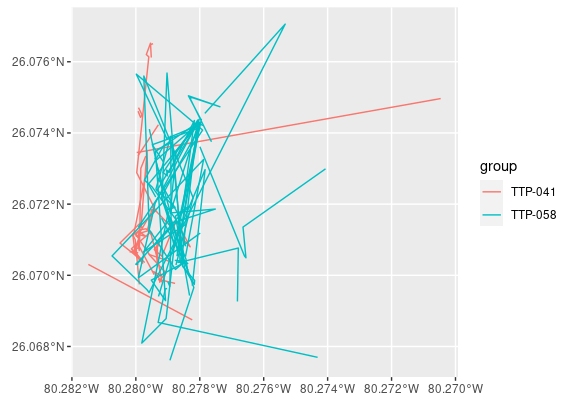You can use geom_sftrack just like any other ggplot layer, which means you can continue to make manuscript quality plots. cols <- c('TTP-041_1'= 'dodgerblue1','TTP-041_2'= 'darkseagreen2', 'TTP-058_1' = 'darkorchid1', 'TTP-058_2' = 'khaki3') ggplot() + geom_sftrack(data = my_sftrack, size=3, alpha = 0.5) + scale_color_manual(values = cols) + ggtitle('Raccoons at Tree Tops Park Winter 2020') + theme_bw() + theme(axis.text.x = element_text(angle = 45, hjust = 1))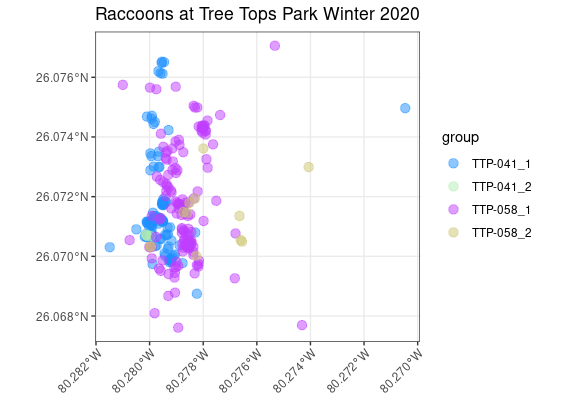## Special functions for working with an sftraj To help with working with more complex sftraj objects, there is a growing suite of sftraj specific functions: ### Step mode in plotting sftraj objects create linestrings for each row of data, as each row is not assumed to be related to the next row of data. This data structure may become inefficient to work with when plotting large data sets. When appropriate these trajectories can be generalized by combining linestrings where lines meet. For plotting purposes we can create these linestrings quickly and plot them at much faster speeds than individual lines. For plot and geom_sftrack there is an argument called step_mode that refers to whether youd like to plot the lines individually (step_mode = T), or generalize them into connected linestrings (step_mode = F). By default step_mode is set to TRUE. library("ggplot2") ggplot() + geom_sftrack(data = my_sftraj, step_mode = TRUE)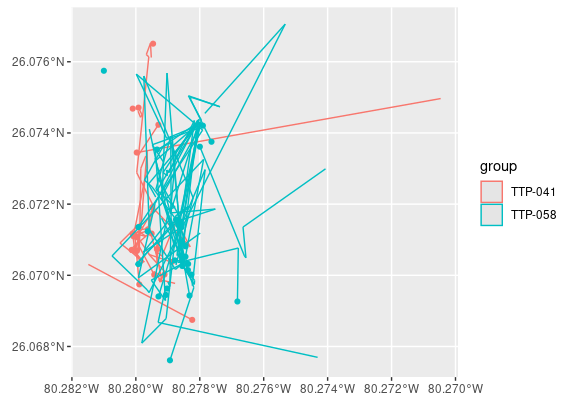You’ll notice that the appearance of the plot changes as the POINTs are also displayed. This is because step mode adds POINTS to the plot that contain a fill as well as a color property. ### coord_traj This function returns a data.frame (x,y,z) of the point at t1 of each sftraj geometry. It works nearly identically to sf::st_coordinates(). coord_traj(my_sftraj$geometry)[1:10,]
##            [,1]     [,2]
##  [1,]        NA       NA
##  [2,] -80.27906 26.06945
##  [3,]        NA       NA
##  [4,]        NA       NA
##  [5,] -80.27431 26.06769
##  [6,] -80.27930 26.06867
##  [7,] -80.27908 26.06962
##  [8,] -80.27902 26.06963
##  [9,]        NA       NA
## [10,] -80.27900 26.06982

### pts_traj

If youd like to retain the geometries but still pull out t1 point you can use pts_traj(). This functions returns a list of the beginning point of each sftraj geometry, or an sfc column when using the argument sfc = TRUE.

pts_traj(my_sftraj$geometry)[1:5] ## [] ## POINT EMPTY ## ## [] ## POINT (-80.27906 26.06945) ## ## [] ## POINT EMPTY ## ## [] ## POINT EMPTY ## ## [] ## POINT (-80.27431 26.06769) pts_traj(my_sftraj$geometry, sfc= TRUE)[1:5]
## Geometry set for 5 features  (with 3 geometries empty)
## Geometry type: POINT
## Dimension:     XY
## Bounding box:  xmin: -80.27906 ymin: 26.06769 xmax: -80.27431 ymax: 26.06945
## CRS:           +proj=longlat +ellps=WGS84 +datum=WGS84 +no_defs
## POINT EMPTY
## POINT (-80.27906 26.06945)
## POINT EMPTY
## POINT EMPTY
## POINT (-80.27431 26.06769)

### is_linestring

May help if you’d like to quickly filter an sftraj object to just contain pure linestrings. is_linestring() returns TRUE or FALSE if the geometry is a linestring. This does not recalculate anything, it just filters out steps that contained NAs in either phase. Its nearly identical to st_is(x,‘LINESTRING’), but may be more intuitive for users.

is_linestring(my_sftraj$geometry)[1:10] ##  FALSE FALSE FALSE FALSE TRUE TRUE TRUE FALSE FALSE TRUE new_sftraj <- my_sftraj[is_linestring(my_sftraj$geometry),]
head(new_sftraj)
## Sftraj with 6 features and 12 fields (0 empty geometries)
## Geometry : "geometry" (XY, crs: +proj=longlat +ellps=WGS84 +datum=WGS84 +no_defs)
## Timestamp : "time" (POSIXct in UTC)
## Grouping : "sft_group" (*id*)
## -------------------------------
##    animal_id latitude longitude           timestamp height hdop vdop fix month
## 5    TTP-058 26.06769 -80.27431 2019-01-19 04:02:30    858  5.1  3.2  2D     1
## 6    TTP-058 26.06867 -80.27930 2019-01-19 05:02:30    350  1.9  3.2  3D     1
## 7    TTP-058 26.06962 -80.27908 2019-01-19 06:02:30     11  2.3  4.5  3D     1
## 10   TTP-058 26.06982 -80.27900 2019-01-19 17:02:30     NA  2.0  3.3  3D     1
## 11   TTP-058 26.06969 -80.27894 2019-01-19 18:02:05      8  4.2  2.5  3D     1
## 12   TTP-058 26.07174 -80.27890 2019-01-19 19:02:04     -3  0.9  1.5  3D     1
##                   time               sft_group                       geometry
## 5  2019-01-19 04:02:30 (id: TTP-058, month: 1) LINESTRING (-80.27431 26.06...
## 6  2019-01-19 05:02:30 (id: TTP-058, month: 1) LINESTRING (-80.2793 26.068...
## 7  2019-01-19 06:02:30 (id: TTP-058, month: 1) LINESTRING (-80.27908 26.06...
## 10 2019-01-19 17:02:30 (id: TTP-058, month: 1) LINESTRING (-80.279 26.0698...
## 11 2019-01-19 18:02:05 (id: TTP-058, month: 1) LINESTRING (-80.27894 26.06...
## 12 2019-01-19 19:02:04 (id: TTP-058, month: 1) LINESTRING (-80.2789 26.071...

### Calculating step metrics of an sftraj

For use in movement models, you may need to calculate the dx, dy, length, and turn angles of each step. You can do that in sftrack using step_metrics(). It should be noted it will accept an sftrack object, however, it first converts the geometries internally to sftraj geometries and then calculates step metrics. As with other sf objects, the return is assumed to be in the units of the crs when not specified. Absolute angle is measured in radians.

step_metrics(my_sftraj[1:10,])
##            dx          dy       dist    dt abs_angle rel_angle       speed
## 1          NA          NA         NA  3600        NA        NA          NA
## 2    0.000000   0.0000000   0.000000  3600        NA        NA 0.000000000
## 3          NA          NA         NA  3600        NA        NA          NA
## 4          NA          NA         NA  3600        NA        NA          NA
## 5  498.309348 109.0329742 510.096380  3600 2.9272914        NA 0.141693439
## 6   22.473674 105.0175956 107.395332  3600 1.3588662 -1.568425 0.029832037
## 7    5.549011   0.9266259   5.625847  3574 0.1645841 -1.194282 0.001574104
## 8    0.000000   0.0000000   0.000000  3626        NA        NA 0.000000000
## 9          NA          NA         NA 32400        NA        NA          NA
## 10         NA          NA         NA    NA        NA        NA          NA
##                     sftrack_id
## 1  TTP-058_2019-01-19 00:02:30
## 2  TTP-058_2019-01-19 01:02:30
## 3  TTP-058_2019-01-19 02:02:30
## 4  TTP-058_2019-01-19 03:02:30
## 5  TTP-058_2019-01-19 04:02:30
## 6  TTP-058_2019-01-19 05:02:30
## 7  TTP-058_2019-01-19 06:02:30
## 8  TTP-058_2019-01-19 07:02:04
## 9  TTP-058_2019-01-19 08:02:30
## 10 TTP-058_2019-01-19 17:02:30`